# Brayton Cycle – Principles and Calculations

#### Engineering

The Brayton cycle is a thermodynamic cycle that utilizes warm air as the working fluid. The cycle sees common use in jet engines, because of the flow of air and thrust the cycle can provide. This cycle allows transforms fuel and flowing air into usable work. In this article, you will learn the principles of the Brayton cycle, the calculation of values, and variations of the cycle.

## Principles of the Brayton Cycle

The Brayton cycle is comprised mainly of a compressor, a combustion chamber, turbine, and a heat exchanger or simple intake/exhaust valve depending on process requirements. The Brayton cycle utilizes air as the working fluid of the system making it only require fuel to function.

The process begins by intaking air through some sort of manifold. The air is then run through a compressor to increase the pressure. Next, the air pushes through a combustion chamber and undergoes an isobaric process where the fuel is combusted, heating up the air. Afterward, the air pushes through the turbine, producing mechanical work. Finally, the air is released through an exhaust valve exiting the system. This cycle is optimal for aircraft because already flowing air serves as the working fluid and the cycle can easily be made in an aerodynamic way.

## Calculations of the Brayton Cycle

When analyzing the Brayton Cycle the goal is to find all the enthalpies to better understand the cycle efficiency. Using these efficiencies helps find the weak points of the cycle and how to use less fuel for more work. Since this cycle relies upon air as the working fluid, there is a pressure ratio that needs to be tracked throughout the cycle. Using the ideal gas law, airflow and properties are able to be fully tracked throughout the cycle.

### Stage 1 ➔ 2 (Compressor)

Typically, temperature (T1) going into the compressor, and the pressure ratio (rp) of the system is predetermined. Air tables can be deployed to find the first enthalpy (h1) and the relative pressure (Pr1).

#### Ideal-

Using the relative pressure and pressure ratios allows determination of relative pressure (Pr2) at the second stage of the cycle.which can be altered into a more usable form.Using the values above and below the relative pressure, interpolation allows determination of the temperature(T2) and enthalpy (h2).#### Actual-

When analyzing a cycle for the actual values there is a compressor efficiency(ηc) that must be taken into Account. A constant is also used that relates pressure and temperature(γ).

First, find the theoretical temperature (T2s) at stage two of the cycle.Next, using the found temperature and the compressor efficiency, the actual temperature(T2) at stage two can be found.Finally, use the actual temperature of stage 2 and find the enthalpy(h2) by interpolation.### Stage 2 ➔ 3 (Combustion Chamber)

Using the desired temperature (T3) after combustion, we can then find the enthalpy (h3) and relative pressure (Pr3).### Stage 3 ➔ 4 (Turbine)

#### Ideal-

Using the values found in the previous step, we can determine the properties at the last stage of the Brayton cycle.

These values are the enthalpy (h4) and the relative pressure (Pr4).Using the new relative pressure to interpolate for the final enthalpy.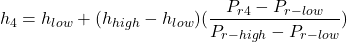#### Actual-

Finding the temperature leaving the turbine and use the turbine efficiency (ηt) and the temperature (T3) going into the turbine.Finally, interpolate for the final enthalpy(h4) value.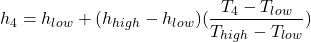### Stage 4 ➔ 1 (Exhaust/Intake or Heat Exchanger)

#### Ideal-

With all values and enthalpies obtained, there are still a few equations that provide full understanding of the cycle.#### Actual-

Use the specific heat of air (Cp) and the temperatures to determine work and heat into and out of the cycle.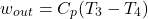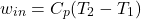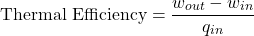## Variations of the Brayton Cycle

### Reheat

Reheating in the Brayton cycle involves pulling air out from the turbine and putting it back into the combustion chamber. The reheated air then runs through a secondary turbine. This process increases the net work of the cycle at a and makes it more efficient in terms of input to output.

### Regeneration

Regeneration in the Brayton cycle uses the hot air exiting the turbine to preheat the air exiting the compressor. This increases the thermal efficiency of the system by reducing waste heat lost to the environment. It also reduces the amount of fuel needed to heat the air.